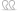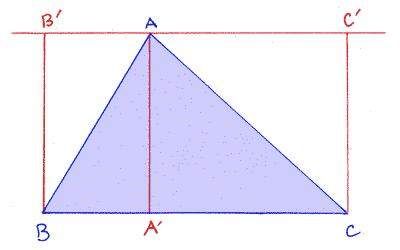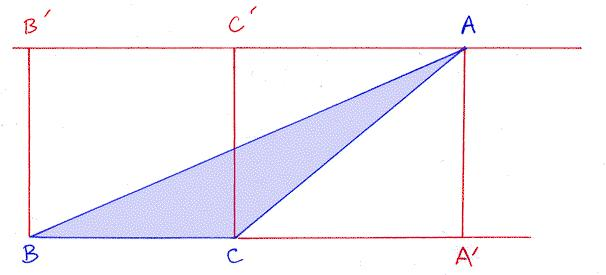# Thread: Question

1. ## Question

How do you work out the square footage of a triangle?Reply With Quote

2. One half the height times the base.Reply With Quote

3. ^ dang - yo beat me to it.

well thread closed.Reply With Quote

4. You mean I was right?Reply With Quote

5.Originally Posted by buadhai
You mean I was right?
Nope, but who cares if he has 900 tiles left overReply With Quote

6.Originally Posted by buadhai
One half the height times the base.
Only if it is a 90 degree triangle.Reply With Quote

7. The Area of a Triangle

The formula for the area of a triangle is:
area = one-half (length of the base) times (length of the the altitude).
You have to use the same linear units (inches, say) to measure the base and the altitude. The area is then given in square units (square inches, if we used inches to measure the sides).
How do you give a reasoned account of the formula, that is, an account that demonstrates why it is the "right way" to find a triangle's area? We cannot begin reasoning without some prior knowledge. We need to base our reasoning on something. We begin, therefore, assuming that we all already know and understand that the area of a rectangle is found by multiplying its length and its width.
Consider how we might determine the area of triangle ABC in the figure below:We've added a line through A that is parallel to the base BC, and we've marked points A', B' and C' to make 3 segments, each of which is perpendicular to BC.
Now the reasoned explanation can go as follows: Triangle ABC covers exactly one-half of the area in rectangle BCC'B'. But the area of this rectangle is |BC| | AA'| = (base of ABC)(altitude of ABC). The area of the triangle is half this.
Area ABC = (1/2) (base of ABC)(altitude of ABC)
All seems well and good. However, this reasoning is not adequate for some triangles. What if A is not over the base? This situation is illustrated in the next picture:In this case, the reasoning has to be different.Reply With Quote

8.Reply With Quote

9. Area of Triangle = 1/2 Base x HeightReply With Quote

10. The area of my GF's triangle is about 3/4 the size of me handReply With Quote

11.Originally Posted by NickA
area = one-half (length of the base) times (length of the the altitude).
I knew I was right....Originally Posted by flash
One half the height times the base.Reply With Quote

12. ok thanks for all that, I have found my own method.

I will buy tiles a plenty and return the unopened boxes.Reply With Quote

13. Not sure if you can get triangular tiles most of them seem
to be rectangular these daysReply With Quote

14. I'll use a lot of grout.Reply With Quote

#### Thread Information

##### Users Browsing this Thread

There are currently 1 users browsing this thread. (0 members and 1 guests)

####Posting Permissions

• You may not post new threads
• You may not post replies
• You may not post attachments
• You may not edit your posts
•